TUTORIALS
TUTORIALS HOME

GENERAL MATH
NUMBER SETS
ABSOLUTE VALUE & INEQUALITIES
SETS & INTERVALS
FRACTIONS
POLYNOMIALS
LINEAR EQUATIONS
GEOMETRY
FINITE SERIES
TRIGONOMETRY
EXPONENTS
LOGARITHMS
INDUCTION

CALCULUS
LIMITS
DERIVATIVES
RELATED RATES & OPTIMIZATION
CURVE SKETCHING
INTEGRALS
AREA & VOLUME
INVERSE FUNCTIONS

MAIN
HOME
TESTS
TUTORIALS
SAMPLE PROBLEMS
COMMON MISTAKES
STUDY TIPS
GLOSSARY
MATH HUMOUR
CALCULUS APPLICATIONS

# Definite Integrals

The formal definition of a definite integral is stated in terms of the limit of a Riemann sum. Riemann sums are covered in the calculus lectures and in the textbook. For simplicity's sake, we will use a more informal definiton for a definite integral. We will introduce the definite integral defined in terms of area.

Let f(x) be a continuous function on the interval [a,b]. Consider the area bounded by the curve, the x-axis and the lines x=a and x=b. The area of the region that lies above the x-axis should be treated as a positive (+) value, while the area of the region that lies below the x-axis should be treated as a negative (-) value.

The image below illustrates this concept. The positive area, above the x-axis, is shaded green and labelled "+", while the negative area, below the x-axis, is shaded red and labelled "-".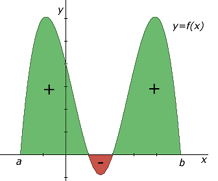The integral of the function f(x) from a to b is equal to the sum of the individual areas bounded by the function, the x-axis and the lines x=a and x=b. This integral is denoted by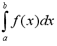where f(x) is called the integrand, a is the lower limit and b is the upper limit. This type of integral is called a definite integral. When evaluated, a definite integral results in a real number. It is independent of the choice of sample points (x, f(x)).

# Properties of Definite Integrals

The following properties are helpful when calculating definite integrals.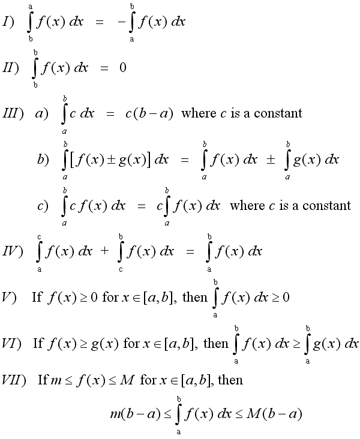# Examples

1 | Evaluate the integral by finding the area beneath the curve

# The Fundamental Theorem of Calculus

The Fundamental Theorem of Calculus defines the relationship between the processes of differentiation and integration. That relationship is that differentiation and integration are inverse processes.

# The Fundamental Theorem of Calculus : Part 1

If f is a continuous function on [a,b], then the function denoted by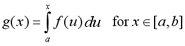is continuous on [a,b], differentiable on (a,b) and g'(x) = f(x).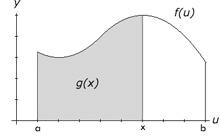If f(t) is continuous on [a,b], the function g(x) that's equal to the the area bounded by the u-axis and the function f(u) and the lines u=a and u=x will be continuous on [a,b] and differentiable on (a,b). Most importantly, when we differentiate the function g(x), we will find that it is equal to f(x). The graph to the right illustrates the function f(u) and the area g(x).

# The Fundamental Theorem of Calculus : Part 2

If f is a continuous function on [a,b], then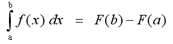where F is any antiderivative of f.

If f is continuous on [a,b], the definite integral with integrand f(x) and limits a and b is simply equal to the value of the antiderivative F(x) at b minus the value of F at a. This property allows us to easily solve definite integrals, if we can find the antiderivative function of the integrand.

Parts one and two of the Fundamental Theorem of Calculus can be combined and simplified into one theorem.

# The Fundamental Theorem of Calculus

Let f be a continuous function on [a,b].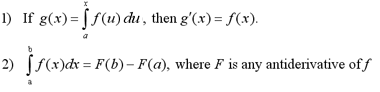# Indefinite Integrals

An indefinite integral has the form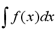When evaluated, an indefinite integral results in a function (or family of functions). An indefinite integral of a function f(x) is also known as the antiderivative of f. A function F is an antiderivative of f on an interval I, if F'(x) = f(x) for all x in I. This is a strong indication that that the processes of integration and differentiation are interconnected.

# Table of Indefinite Integrals

The following tables list the formulas for antidifferentiation. These formulas allow us to determine the function that results from an indefinite integral. Since the formulas are for the most general indefinite integral, we add a constant C to each one. With these formulas and the Fundamental Theorem of Calculus, we can evaluate simple definite integrals.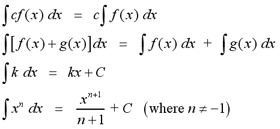The next table lists indefinite integrals involving trigonometric functions.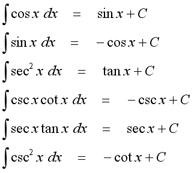Note: After finding an indefinite integral, you can always check to see if your answer is correct. Since integration and differentiation are inverse processes, you can simply differentiate the function that results from integration, and see if it is equal to the integrand.

# Examples

2 | Find the general indefinite integrals
3 | Evaluate the definite integral
4 | Evaluate the definite integral of the absolute value of a function

# The Total Change Theorem

The total change theorem is an adaptation of the second part of the Fundamental Theorem of Calculus. The Total Change Theorem states: the integral of a rate of change is equal to the total change.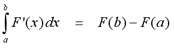If we know that the function f(x) is the derivative of some function F(x), then the definite integral of f(x) from a to b is equal to the change in the function F(x) from a to b.

# Examples

5 | Given the velocity function, find the displacement during a period of time

# The Substitution Rule

Suppose that we have an integral such as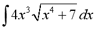With our current knowledge of integration, we can't find the general equation of this indefinite integral. There are no antidifferentiation formulas for this type of integral. However, from our knowledge of differentiation, specifically the chain rule, we know that 4x3 is the derivative of the function within the square root, x4 + 7. We must also account for the chain rule when we are performing integration. To do this, we use the substitution rule.

The Substitution Rule states: if u = g(x) is a differentiable function and f is continuous on the range of g, then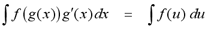Note: Recall that if u = g(x), then du = g'(x)dx. If we substitute u into the left side of the equation for g(x) and du for g'(x)dx, then we get the integral on the right side of the equation.

From our previous example, if we let u = (x4+7), then du = 4x3dx. If we substitutite these values into the integral, we get an integral that can be solved using the antidifferentiation formulas.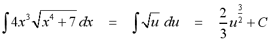However, this answer is still in terms of u. We must substitute u = (x4+7) into the resulting function, so that it is a function of x, rather than u.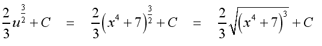The substitution rule also applies to definite integrals. The Substitution Rule for Definite Integrals states: If f is continuous on the range of u = g(x) and g'(x) is continuous on [a,b], then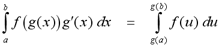# Examples

6 | Find the general indefinite integrals using the substitution rule
7 | Evaluate the definite integral using the substitution rule

# Integrals of Symmetric Functions

If f(x) is continuous on [-a, a] and f is an even function, then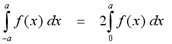If f(x) is continuous on [-a, a] and f is an odd function, then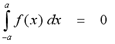These properties of integrals of symmetric functions are very helpful when solving integration problems. Some of the more challenging problems can be solved quite simply by using this property.

# Examples

8 | Evaluate the definite integral of the symmetric function

# Integration By Parts

Suppose that we have an integral such as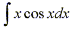Similar to integrals solved using the substitution method, there are no general equations for this indefinite integral. However there do not appear to be any clear substitutions that could be made to simplify this integral. This brings us to an integration technique known as integration by parts, which will call upon our knowledge of the Product Rule for differentiation.

The Product Rule states: If f and g are differentiable functions, then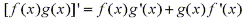By taking the indefinite integral of both sides of the equation we have: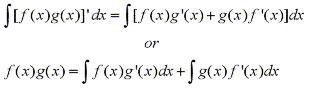and we can rearrange this equation as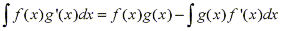To make it easier to remember it is commonly written in the following notation. Let u=f(x) and v=g(x). Then the differentiables are du=f'(x)dx and dv=g'(x)dx, so by the substitution rule, the formula for integration by parts becomes: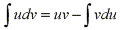From our previous example, if we let u=x and dv=cosx, then du=dx and v=sinx. If we substitute these values into the formula we have: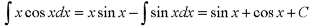Note: By choosing u=x we obtain a simpler integral than we started with. Had we chose u=cosx and dv=x then du=-sinx and v=(1/2)x2 so integration by parts gives: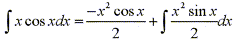This equation is correct, but the integral is more difficult than the one we started with.

When choosing u and dv always try to choose u=f(x) to be a function that becomes simpler when differentiated (or at least not more complicated) and to choose dv=g'(x) to be a function that can be easily integrated to give v.

# Examples

9 | Find the general indefinite integral by integration by parts
10 | Evaluate the definite integral by integration by parts

# Trigonometric Integrals

Suppose we have an integral such as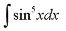The easy mistake is to simply make the substitution u=sinx, but then du=cosxdx. So in order to integrate powers of sine we need an extra cosx factor. Similarily, in order to integrate powers of cosine we need an extra sinx factor. Thus for this example knowing we need an extra sinx factor to integrate powers of cosine we can separate one sine factor and convert the remaining sin4x to an expression involving cosine using the identity sin2x + cos2x = 1.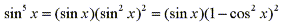Now by using our knowledge of substitution we can evaluate the integral by letting u=cosx, then du=-sinxdx and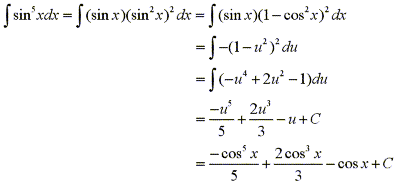Now consider the integral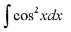If we were to use the method from the previous example and separate one cosine factor we would be left with a factor of cosine of odd degree which isn't easily converted to sine. We must now consider the half angle formulas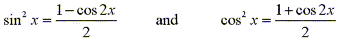Using the half angle formula for cos2x, we have: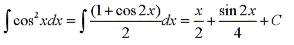# Strategy for Evaluating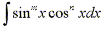(a)
If the power of sine is odd (m=2k+1), save one sine factor and use the identity sin2x + cos2x = 1 to convert the remaining factors in terms of cosine.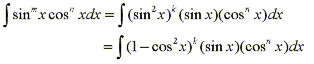then substitute u=cosx.
(b)
If the power of cosine is odd (n=2k+1), save one cosine factor and use the identity sin2x + cos2x = 1 to convert the remaining factors in terms of sine.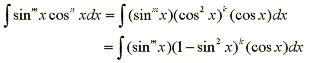then substitute u=sinx.
(c)
If the powers of both sine and cosine are even then use the half angle identities.In some cases it may be helpful to use the identity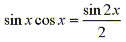11 | Find the indefinite trigonometric integral
12 | Using the half angle formulas solve the indefinite trigonometric integral
13 | Find the definite trigonometric integral

Now that we have learned strategies for solving integrals with factors of sine and cosine we can use similar techniques to solve integrals with factors of tangent and secant. Using the identity sec2x = 1 + tan2x we are able to convert even powers of secant to tangent and vice versa. Now we will consider two examples to illustrate two common strategies used to solve integrals of the form

Suppose we have an integral such as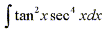Observing that (d/dx)tanx=sec2x we can separate a factor of sec2x and still be left with an even power of secant. Using the identity sec2x = 1 + tan2x we can convert the remaining sec2x to an expression involving tangent. Thus we have: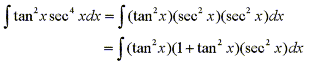Then substitute u=tanx to obtain: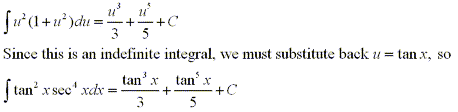Note: Suppose we tried to use the substitution u=secx, then du=secxtanxdx. When we separate out a factor of secxtanx we are left with an odd power of tangent which is not easily converted to secant.

Consider the integral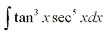Since (d/dx)secx=secxtanx we can separate a factor of secxtanx and still be left with an even power of tangent which we can easily convert to an expression involving secant using the identity sec2x = 1 + tan2x. Thus we have: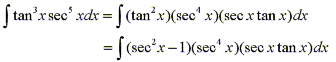Then substitute u=secx to obtain: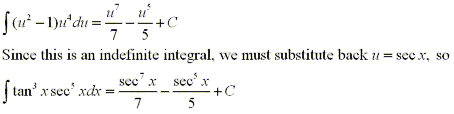Note: Suppose we tried to use the substitution u=tanx, then du=sec2xdx. When we separate out a factor of sec2x we are left with an odd power of secant which is not easily converted to tangent.

# Strategy for Evaluating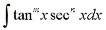(a)
If the power of secant is even (n=2k, k>2) save a factor of sec2x and use the identity sec2x = 1 + tan2x to express the remaining factors in terms of tanx.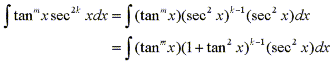then substitute u=tanx.
(b)
If the power of tangent is odd (m=2k+1), save a factor of secxtanx and use the identity sec2x = 1 + tan2x to express the remaining factors in terms of secx.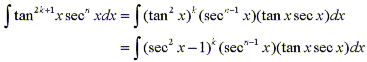then substitute u=secx.

Note: If the power of secant is even and the power of tangent is odd then either method will suffice, although there may be less work involved to use method (a) if the power of secant is smaller, and method (b) if the power of tangent is smaller.

14 | Find the indefinite trigonometric integral
15 | Find the definite trigonometric integral

Integrals of cotangent and cosecant are very similar to those with tangent and secant.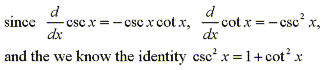it is easy to see that integrals of the form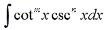can be solved by nearly identical methods as are integrals of the form.

16 | Find the indefinite trigonometric integral

Unlike integrals with factors of both tangent and secant, integrals that have factors of only tangent, or only secant do not have a general strategy for solving. Use of trig identities, substitution and integration by parts are all commonly used to solve such integrals. For example,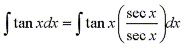If we make the substitution u=secx, then du=secxtanxdx, and we are left with the simple integral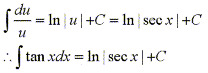Similarily we can use the same technique to solve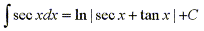17 | Find the definite trigonometric integral
18 | Find the definite trigonometric integral
19 | Find the indefinite trigonometric integral

Another problem that may be encountered when solving trigonometric integrals are integrals of the form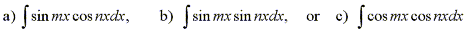Using the product formulas which are deduced from the addition/subtraction rules we have the corresponding identities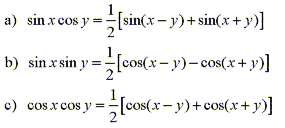20 | Find the indefinite trigonometric integral using the product formulas

# Trigonometric Substitution

Sometimes trigonometric substitutions are very effective even when at first it may not be so clear why such a substitution be made. For example, when finding the area of a circle or an ellipse you may have to find an integral of the form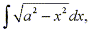where a>0.

It is difficult to make a substitution where the new variable is a function of the old one, (for example, had we made the substitution u = a2 - x2, then du= -2xdx, and we are unable to cancel out the -2x.) So we must consider a change in variables where the old variable is a function of the new one. This is where trigonometric identities are put to use. Suppose we change the variable from x to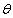by making the substitution x = a sin θ. Then using the trig identity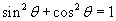we can simplify the integral by eliminating the root sign.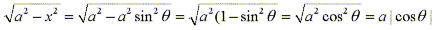By changing x to a function with a different variable we are essentially using the The Substitution Rule in reverse. If x=g(t) then by restricting the boundaries on g we can assure that g has an inverse function; that is, g is one-to-one. In the example above we would require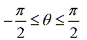to assure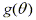has an inverse function.

If we look at the Substitution Rule and replace u with x and x with t, we obtain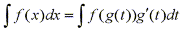This is known as the "inverse substitution".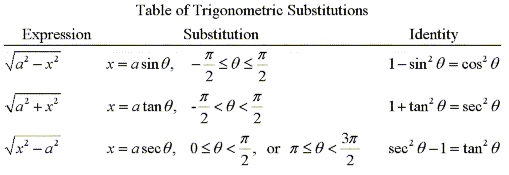# Integration of Rational Functions By Partial Fractions

Integration of rational functions by partial fractions is a fairly simple integrating technique used to simplify one rational function into two or more rational functions which are more easily integrated.

Think back to the steps taken when adding or subtracting fractions that do not have the same denominator. First you find the lowest common multiple of the two denominators and then cross multiply with the numerators accordingly. eg.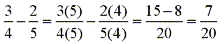Well the same process applies when dealing with polynomial fractions. eg.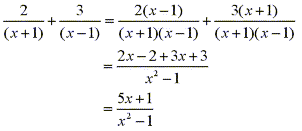Now by reversing this process we can simplify a function such as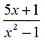into two fractions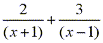which are more easily integrated.

This process is possible when the function is proper; that is the degree of the numerator is less than the degree of the denominator. If the function is improper; that is the degree of the numerator is greater than or equal to the degree of the denominator, then we must first use long division to divide the denominator into the numerator until we obtain a remainder, such that it's degree is less than the denominator. Then if possible the above process is used to simplify the proper function.

To complete some of the problems in this section it will be useful to know the table integral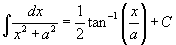In general there are 4 cases to consider to express a rational function as the sum of two or more partial fractions.

Case 1
The denominator is a product of distinct linear factors (no factor is repeated or a constant mulptiple of another).

For example,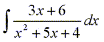Since the degree of the numerator is less than the degree of the denominator we don't need to divide. The denominator can be factored as follows: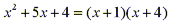Since the denominator has distinct linear factors we can write the rational fraction as the sum of two or more partial fractions as follows: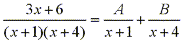By multiplying both sides by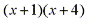we have: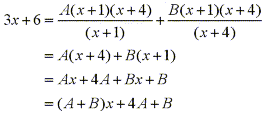From this equation we can match terms of the same degree to determine the coefficients by solving the following system of equations: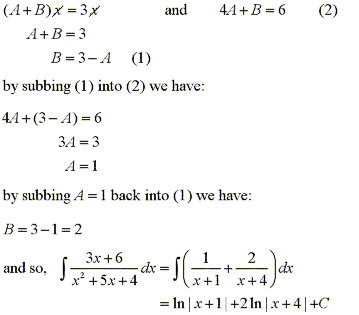Case 2

The denominator is a product of linear functions, some of which are repeated.

For example,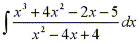Since the degree of the numerator is greater than the degree of the denominator we must factorize by long division.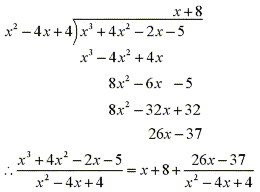So we can now factor the denominator to obtain: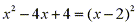Since the linear factor (x-2) occurs twice, the partial fraction decomposition is: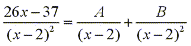When we multiply both sides by the least common denominator we get: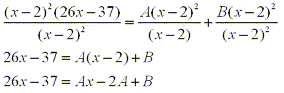From this equation we can match terms of the same degree to determine the coefficients by solving the following system of equations: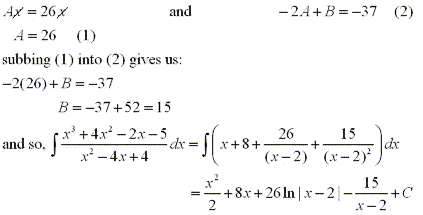Case 3

The denominator contains irreducible quadratic factors, none of which are repeated.

When reducing such functions to partial fractions if there is a term in the denominator of the form ax2 + bx + c, where b2 - 4ac < 0, then the numerator for that partial fraction will be of the form Ax + B.

For example,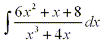Since the degree of the numerator is less than the degree of the denominator we do not have to divide first.

Since x3 + 4x = x(x2 + 4) can't be factored any further we have: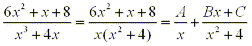multiplying both sides by x(x2 + 4), we have: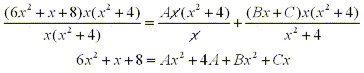From this equation we can match terms of the same degree to determine the coefficients by solving the following system of equations: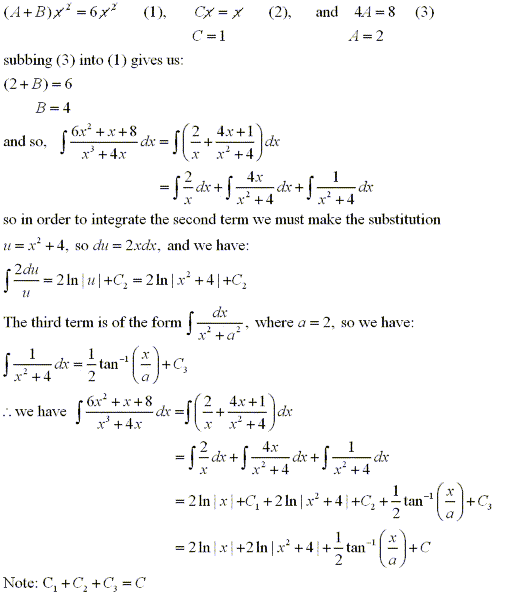Case 4

The denominator contains a repeated irreducible quadtratic factor.

Functions of this form are the same as those in case 3 only there is a term in the denominator that is repeated or is a constant multiple of another.

For example,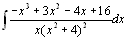If we were to expand the denominator we would see that its degree is greater than the the degree of the numerator so we do not have to divide first.

Since the function cannot be factored any further we have: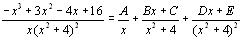multiplying both sides by (x + 1)(x2 + 4)2, we have: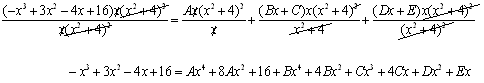From this equation we can match terms of the same degree to determine the coefficients by solving the following system of equations: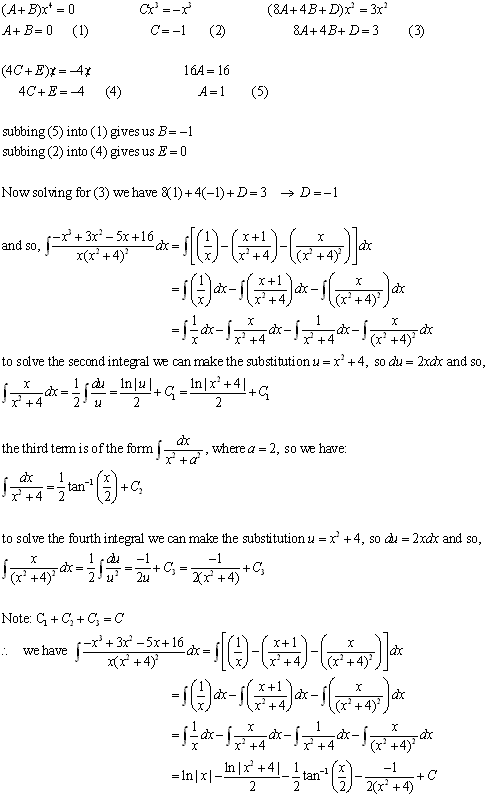# Improper Integrals

In all of the previous tutorials we have dealt with integrals with a continous function f on a finite interval [a,b]. In this section we will consider two types of integrals known as improper integrals. The first type of improper integral are those defined on an infinite interval, and the second are those where the function f has an infinite discontinuity in [a,b].

Type 1: Infinite Intervals

Type 2: Discontinous Integrands

For more practice with the concepts covered in this tutorial, visit the Integral Problems page at the link below. The solutions to the problems will be posted after these chapters are covered in your calculus course.

To test your knowledge of integration problems, try taking the general integrals test on the iLrn website or the advanced integrals test at the link below.

Integral Problems
General Integrals Test on iLrn

|Top of Page |

COURSE HOMEPAGES
MATH 1036
MATH 1037

FACULTY HOMEPAGES
Alex Karassev
Ted Chase